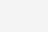# 高中数学函数性质-单调性与奇偶性

1、定义法：利用定义证明函数单调性的一般步骤是：①任取x1、x2∈D，且x1<x2;

②作差f(x1)-f(x2)，并适当变形(“分解因式”、配方成同号项的和等);

2、导数法：

a.若使得f′(x)=0的x的值只有有限个，则如果f ′(x)≥0，则f(x)在区间D内为增函数;如果f′(x) ≤0，则f(x)在区间D内为减函数。

b.单调性的判断方法：定义法及导数法、图象法、复合函数的单调性(同增异减)、用已知函数的单调性等。

1、若f(x)，g(x)均为增(减)函数，则f(x)+g(x)仍为增(减)函数。

2、互为反函数的两个函数有相同的单调性。

3、y=f[g(x)]是定义在M上的函数，若f(x)与g(x)的单调性相同，则其复合函数f[g(x)]为增函数;若f(x)、g(x)的单调性相反，则其复合函数f[g(x)]为减函数，简称”同增异减”。

4、奇函数在关于原点对称的两个区间上的单调性相同;偶函数在关于原点对称的两个区间上的单调性相反。

1、图象的对称性质：

2、设f(x)，g(x)的定义域分别是D1，D2那么在它们的公共定义域上：奇+奇=奇，奇×奇=偶，偶+偶=偶，偶×偶=偶，奇×偶=奇

3、任意一个定义域关于原点对称的函数f(x)均可写成一个奇函数g(x)与一个偶函数h(x)和的形式，则4、奇偶函数图象的对称性

(1)若y=f(a+x)是偶函数，则f(a+x)=f(a-x)↔f(2a-x)=f(x)↔f(x)的图象关于直线x=a对称;(2)若y=f(b+x)是偶函数，则f(b-x)=-f(b+x)↔f(2a-x)=-f(x)↔f(x)的图象关于点(b,0)中心对称

5、一些重要类型的奇偶函数：(0)

2.3K0

8160

6740